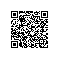# python光荣之路测试开发班list学习笔记

# coding=utf-8

book_list_in_library = []
borrowed_book_list = []

input 1:add new book to library
input 2:borrow book from library
input 3:list all books in library
input 4:list all borrowed books in library
input 5: list current books in library
input 6:lend a book
"""

global book_list_in_library
book_list_in_library.append(book_name)

def borrow_book():
global borrowed_book_list
while 1:
book_name = raw_input("please input the book name to borrow:")
borrowed_book_list.append(book_name)
print "borrow book done!"
break
else:
print "the book you borrow does not exist,try again!"

def lend_book():
global borrowed_book_list
while 1:
book_name = raw_input("please input the book name to lend:")
if book_name in borrowed_book_list:
borrowed_book_list.remove(book_name)
print "lend book done!"
break
else:
print "the book you lend does not exist,try again!"

while 1:
if command == "1":
print book_list_in_library
continue
if command == "2":
borrow_book()
if command == "3":
print "all books are :", book_list_in_library
if command == "4":
print "borrowed books are :", borrowed_book_list
if command == "5":
print "current books in library are :", ready_borrow_book_list
if command == "6":
lend_book()
if command == "q":
break使用钉钉扫一扫加入圈子
+ 订阅